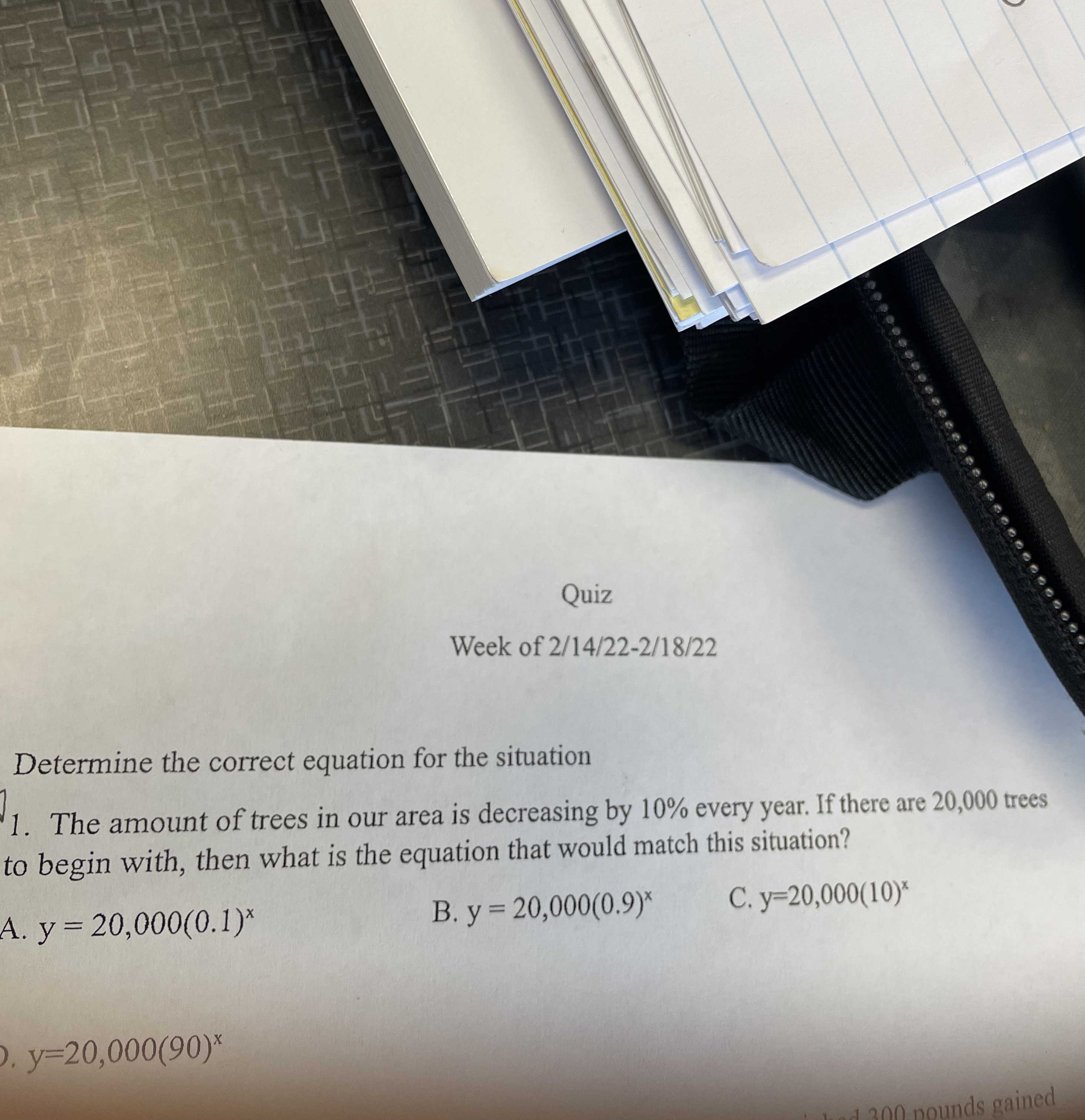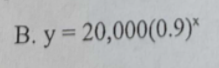### ¿Todavía tienes preguntas de matemáticas?

Pregunte a nuestros tutores expertos
Algebra
Pregunta1. The amount of trees in our area is decreasing by $$10 \%$$ every year. If there are $$20,000$$ trees to begin with, then what is the equation that would match this situation?

A. $$y = 20,000 ( 0.1 ) ^ {x }$$

B. $$y = 20,000 ( 0.9 ) ^ { x }$$

C. $$y = 20,000 ( 10 ) ^ { x }$$

D. $$y = 20,000 ( 90 ) ^ { x }$$